###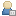Show Posts

This section allows you to view all posts made by this member. Note that you can only see posts made in areas you currently have access to.

### Topics - Dr_D

Pages: 
1
##### General coding questions / Aligning a matrix with a polygon normal(axis-angle)
« on: August 25, 2011 »
Hey guys. I'm having a matrix problem here. I'm using FreeBASIC, but as this is a general math problem I put it here. Anyway... here we go. I need to align a 3d object with a polygon normal. To be more precise, I need to align the object's up vector with the polygon normal, while still allowing free rotation about it. In general this isn't a problem... if the current rotation about the up vector isn't important. For what I'm trying to do here here though, it is.

For instance, I can copy the polygon's normal to the up vector. I can then subtract the midpoint from one of the polygon's vertices and normalize to get the right vector . Then I can take the cross product of those two to get the forward vector and complete an orthogonal rotation matrix. All that sounds fine... but not when you're simulating a vehicle. I need to be able to rotate freely about up and keep the angle consistent when a new collision is detected. I tried with an axis-angle approach, but something seems to be off. Has anyone ever dealt with this before? I've been testing different methods for about a week now and it's starting to drive me nuts. :p

2
##### Freebasic / Clip coordinates problem... or to put it better, the lack thereof.
« on: October 12, 2010 »
Ok, so I've been following the math on this page, and trying to recreate it all on the CPU, even as will be exponentially slower. I'm not concerened about that, I'm just trying to become a better all around graphics programmer. Anyway, I don't understand exactly how they're handling the clip coordinates. What I'm doing... is simply missing something, as I have vertices that are being projected to infinity, where there is no chance to clip those when they are close to the eye, as they are wildly out of range. Here is some code... if that may help anyone help me. Thanks guys.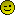Code: [Select]
`   dim as single w = any dim as vec4f pvec  'clip coordinates dim as vec3f ndvec 'normalized device coordinates for i as integer = 0 to Model->max_vertices - 1 with model->tvertices[i] lx = .x ly = .y lz = .z end with 'generating clip coordinates here... doesn't seem quite clear to me...                'mar() = view matrix * model matrix with pvec .x = lx*mar(0) + ly*mar(4) + lz*mar(8) + mar(12) .y = lx*mar(1) + ly*mar(5) + lz*mar(9) + mar(13) .z = lx*mar(2) + ly*mar(6) + lz*mar(10) + mar(14) .w = lx*mar(3) + ly*mar(7) + lz*mar(11) + mar(15) end with                                'just keeping the reciprocal under control... if pvec.w > 0 then w = 1f / pvec.w else w = 1f end if 'normalized device coordinates ndvec.x = pvec.x * w ndvec.y = pvec.y * w ndvec.z = pvec.z * w 'screen coordinates calculated now and stored back in model's pvertices array                'everything is still raw in here for clarity and my own sanity...                'tscrh = screen width, tscrh = screen height with model->pvertices[i] .x = (tscrw * ndvec.x) + (ndvec.x + tscrw) .y = top-((tscrh * ndvec.y) + (ndvec.y + tscrh)) .z = ((zFar-zNear)/2) * ndvec.z + ((zFar+zNear)/2) end with next`

3
##### Freebasic / Perspective correct texture mapping problems...
« on: January 25, 2010 »
Hey guys. I was just messing around, and I'm having some problems here. Can anyone spot what I'm doing wrong? It could use tons and tons of optimizations, but I can't get the texture coords working properly now... I don't know what I've done. Thanks!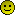Code: [Select]
`#include "fbgfx.bi"type vec2f    as single x, yend typetype vec3f    as single x, y, zend typetype trgb    as uinteger r,g,bend typeconst as integer scr_w = 640const as integer scr_h = 480screenres scr_w, scr_h, 32,,FB.GFX_HIGH_PRIORITYdeclare sub tgpcTri ( byref dst as FB.IMAGE ptr = 0, byval p1 as vec3f, byval t1 as vec2f, byval cl1 as trgb, byval p2 as vec3f, byval t2 as vec2f, byval cl2 as trgb, byval p3 as vec3f, byval t3 as vec2f, byval cl3 as trgb, byref texture as FB.IMAGE ptr )   dim as FB.IMAGE ptr image = imagecreate( 256, 256 )if bload( "rock.bmp", image) <> 0 then    for y as integer = 0 to 255        for x as integer = 0 to 255            dim as integer rgbc = (x and y)            pset image,(x,y), rgb( rgbc, rgbc, rgbc )        next    nextend ifdim as vec3f p1, p2, p3dim as vec2f t1, t2, t3dim as trgb c1, c2, c3t1 = type( 0, 0 )t2 = type( 0, 255 )t3 = type( 255, 255 )c1= type(255,0,0)c2= type(0,255,0)c3= type(0,0,255)do    dim as double ttime = timer    p1 = type( 320+350*sin(ttime), 240+350*cos(ttime), -10 )    p2 = type( 320+350*sin(ttime+1.5), 240+350*cos(ttime+1.5), -10 )    p3 = type( 320+350*sin(ttime+3.14), 240+350*cos(ttime+3.14), 0 )    screenlock    line(0,0)-(640,480),0,bf        tgpcTri(0, p1, t1, c1, p2, t2, c2, p3, t3, c3, image )    screensync    screenunlock        sleep 3,1    loop until inkey\$ <> ""sub tgpcTri ( byref dst as FB.IMAGE ptr = 0, byval p1 as vec3f, byval t1 as vec2f, byval cl1 as trgb, byval p2 as vec3f, byval t2 as vec2f, byval cl2 as trgb, byval p3 as vec3f, byval t3 as vec2f, byval cl3 as trgb, byref texture as FB.IMAGE ptr )           static as uinteger ptr dstptr    static as uinteger ptr srcptr        static as integer x1, x2, x3    static as integer y1, y2, y3    static as integer z1, z2, z3    static as integer u1, u2, u3    static as integer v1, v2, v3    static as integer r1, r2, r3    static as integer g1, g2, g3    static as integer b1, b2, b3    static as integer dw,dh,dbpp,dpitch    static as integer sw,sh,sbpp,spitch    static as integer scanlen, pu, pv    static as integer dx1, dx2, dx3     static as integer dy1, dy2, dy3    static as integer dz1, dz2, dz3    static as integer du1, du2, du3    static as integer dv1, dv2, dv3    static as integer dr1, dr2, dr3    static as integer dg1, dg2, dg3    static as integer db1, db2, db3    static as integer col, r, g, b    static as single sx, ex, sz, ez, su, eu, sv, ev, sr, er, sg, eg, sb, eb    static as single xd1, xd2, xd3, lx, rx, recz    static as single zd1, zd2, zd3, lz, rz, nz, zinc    static as single ud1, ud2, ud3, lu, ru, nu, uinc    static as single vd1, vd2, vd3, lv, rv, nv, vinc    static as single rd1, rd2, rd3, lr, rr, nr, rinc    static as single bd1, bd2, bd3, lb, rb, nb, binc    static as single gd1, gd2, gd3, lg, rg, ng, ginc        if dst = 0 then        dstptr = screenptr        screeninfo dw, dh,, dbpp, dpitch     else        dstptr = cast (uinteger ptr, dst+1 )        dw = dst->width        dh = dst->height        dbpp = dst->bpp        dpitch = dst->pitch    end if    srcptr =  cast (uinteger ptr, texture+1 )    sw = texture->width    sh = texture->height    sbpp = texture->bpp    spitch = texture->pitch               x1 = p1.x    x2 = p2.x    x3 = p3.x        y1 = p1.y    y2 = p2.y    y3 = p3.y        z1 = p1.z    z2 = p2.z    z3 = p3.z    u1 = t1.x    u2 = t2.x    u3 = t3.x    v1 = t1.y    v2 = t2.y    v3 = t3.y        r1 = cl1.r    r2 = cl2.r    r3 = cl3.r        g1 = cl1.g    g2 = cl2.g    g3 = cl3.g        b1 = cl1.b    b2 = cl2.b    b3 = cl3.b    if y2 < y1 then        swap x1, x2        swap y1, y2        swap z1, z2        swap u1, u2        swap v1, v2        swap r1, r2        swap g1, g2        swap b1, b2    end if        if y3 < y1 then        swap x3, x1        swap y3, y1        swap z3, z1        swap u3, u1        swap v3, v1        swap r3, r1        swap g3, g1        swap b3, b1    end if        if y3 < y2 then        swap x3, x2        swap y3, y2        swap z3, z2        swap u3, u2        swap v3, v2        swap r3, r2        swap g3, g2        swap b3, b2    end if        dx1 = x2 - x1    dy1 = y2 - y1    dz1 = z2 - z1    du1 = u2 - u1    dv1 = v2 - v1    dr1 = r2 - r1    dg1 = g2 - g1    db1 = b2 - b1    if dy1 <> 0 then        xd1 = dx1 / dy1        zd1 = dz1 / dy1        ud1 = du1 / dy1        vd1 = dv1 / dy1        rd1 = dr1 / dy1        gd1 = dg1 / dy1        bd1 = db1 / dy1    else        xd1 = 0        zd1 = 0        ud1 = 0        vd1 = 0        rd1 = 0        gd1 = 0        bd1 = 0    end if        dx2 = x3 - x2    dy2 = y3 - y2    dz2 = z3 - z2    du2 = u3 - u2    dv2 = v3 - v2    dr2 = r3 - r2    dg2 = g3 - g2    db2 = b3 - b2    if dy2 <> 0 then        xd2 = dx2 / dy2        zd2 = dz2 / dy2        ud2 = du2 / dy2        vd2 = dv2 / dy2        rd2 = dr2 / dy2        gd2 = dg2 / dy2        bd2 = db2 / dy2    else        xd2 = 0        zd2 = 0        ud2 = 0        vd2 = 0        rd2 = 0        gd2 = 0        bd2 = 0    end if        dx3 = x1 - x3    dy3 = y1 - y3    dz3 = z1 - z3    du3 = u1 - u3    dv3 = v1 - v3    dr3 = r1 - r3    dg3 = g1 - g3    db3 = b1 - b3    if dy3 <> 0 then        xd3 = dx3 / dy3        zd3 = dz3 / dy3        ud3 = du3 / dy3        vd3 = dv3 / dy3        rd3 = dr3 / dy3        gd3 = dg3 / dy3        bd3 = db3 / dy3    else        xd3 = 0        zd3 = 0        ud3 = 0        vd3 = 0        rd3 = 0        gd3 = 0        bd3 = 0    end if        lx = x1    rx = x1    lz = z1    rz = z1    lu = u1    ru = u1    lv = v1    rv = v1    lr = r1    rr = r1    lg = g1    rg = g1    lb = b1    rb = b1        for y as integer = y1 to y2 - 1                if y>-1 and y<dh then                        sx = lx            ex = rx            su = lu            eu = ru            sv = lv            ev = rv            sz = lz            ez = rz            sr = lr            er = rr            sg = lg            eg = rg            sb = lb            eb = rb                        if sx>ex then                swap sx, ex                swap su, eu                swap sv, ev                swap sz, ez                swap sr, er                swap sg, eg                swap sb, eb            end if                        scanlen = (ex-sx)            uinc = (eu-su) / scanlen            vinc = (ev-sv) / scanlen            zinc = (ez-sz) / scanlen            rinc = (er-sr) / scanlen            ginc = (eg-sg) / scanlen            binc = (eb-sb) / scanlen            nu = su/sz            nv = sv/sz            nz = 1.0/sz            nr = sr            ng = sg            nb = sb            for x as integer = sx to ex                                            if x>-1 and x<dw then                    recz = 1.0/nz                                        pu = abs(nu*recz) MOD sw                    pv = abs(nv*recz) MOD sh                    col = *cast(uinteger ptr, cast( ubyte ptr, srcptr) + pv * spitch + pu * sbpp )                    r = (col shr 16) and 255                    g = (col shr 8) and 255                    b = (col)  and 255                    r+=(nr)                    if r<0 then                        r = 0                    elseif r>255 then                         r = 255                    end if                    g+=(ng)                    if g<0 then                        g = 0                    elseif g>255 then                         g = 255                    end if                    b+=(nb)                    if b<0 then                        b = 0                    elseif b>255 then                         b = 255                    end if                    *cast(uinteger ptr, cast( ubyte ptr, dstptr) + y * dpitch + x * dbpp ) = rgb(r,g,b)                                    end if                                nu+=uinc                nv+=vinc                nz+=zinc                nr+=rinc                ng+=ginc                nb+=binc                            next                    end if                lx += xd1        rx += xd3        lu += ud1        ru += ud3        lv += vd1        rv += vd3        lz += zd1        rz += zd3        lr += rd1        rr += rd3        lg += gd1        rg += gd3        lb += bd1        rb += bd3    next            lx = x2    lz = z2    lu = u2    lv = v2    lr = r2    lg = g2    lb = b2    for y as integer = y2 to y3                if y>-1 and y<dh then                        sx = lx            ex = rx            su = lu            eu = ru            sv = lv            ev = rv            sz = lz            ez = rz            sr = lr            er = rr            sg = lg            eg = rg            sb = lb            eb = rb                        if sx>ex then                swap sx, ex                swap su, eu                swap sv, ev                swap sz, ez                swap sr, er                swap sg, eg                swap sb, eb            end if                        scanlen = (ex-sx)+1            uinc = (eu-su) / scanlen            vinc = (ev-sv) / scanlen            zinc = (ez-sz) / scanlen            rinc = (er-sr) / scanlen            ginc = (eg-sg) / scanlen            binc = (eb-sb) / scanlen            nu = su/sz            nv = sv/sz            nz = 1.0/sz            nr = sr            ng = sg            nb = sb                        for x as integer = sx to ex                                if x>-1 and x<dw then                    recz = 1.0/nz                                        pu = abs(nu*recz) MOD sw                    pv = abs(nv*recz) MOD sh                                        col = *cast(uinteger ptr, cast( ubyte ptr, srcptr) + pv * spitch + pu * sbpp )                    r = (col shr 16) and 255                    g = (col shr 8) and 255                    b = (col)  and 255                                        r+=(nr)                    if r<0 then                        r = 0                    elseif r>255 then                         r = 255                    end if                    g+=(ng)                    if g<0 then                        g = 0                    elseif g>255 then                         g = 255                    end if                    b+=(nb)                    if b<0 then                        b = 0                    elseif b>255 then                         b = 255                    end if                    *cast(uinteger ptr, cast( ubyte ptr, dstptr) + y * dpitch + x * dbpp ) = rgb(r,g,b)'*cast(uinteger ptr, cast( ubyte ptr, srcptr) + pv * spitch + pu * sbpp )                                    end if                                nu+=uinc                nv+=vinc                nz+=zinc                nr+=rinc                ng+=ginc                nb+=binc            next                                end if                lx += xd2        rx += xd3        lu += ud2        ru += ud3        lv += vd2        rv += vd3        lz += zd2        rz += zd3        lr += rd2        rr += rd3        lg += gd2        rg += gd3        lb += bd2        rb += bd3    next    end sub`

4
##### Freebasic / Here's something I've been playing with...
« on: December 21, 2009 »
I always seem to forget about this forum from time to time. I don't know why. This place is cool. Sorry.  :p

Anyway.. I've been working on a physics puzzle game for a while. It uses FreeBASIC, OpenGL and Chipmunk. There is an instruction manual(sort of) included.

5
##### Freebasic / This was my first game. :)
« on: March 16, 2009 »
Of course, it wasn't the first thing I ever programmed, but it was the first game I ever made public. Low and behold, Checkers! lol It was originally in QB4.5, but with just a little effort, I ported to FreeBASIC.  It was lost for years on an old CD. I wouldn't even bother looking at the code... it's a total mess of not knowing what to do and trying things out.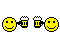6
##### Freebasic / Here's a rotozoomer...
« on: February 08, 2009 »
I don't know if anyone needs it or not, but most of it's programmed inline x86 asm. There is a known bug when drawing to another fb.image, but we'll get that fixed asap.rotozoom

7
##### Challenges & Competitions / [PROCEDURAL] glsl_plasma
« on: April 29, 2008 »
I decided to make another entry since my other one sucked.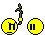This one isn't really anything special either, but I think it's pretty fun to do old-school effects with glsl.

EDIT: I was just informed by a couple of guys with nVidia 8800GTX cards that this just presents a blank screen in Vista. Can anyne confirm that this works on any other card in Vista?

EDIT: Removed old version and added one that should be more compatible with ATI cards.

8
##### Freebasic / Here's an older demo I made using OpenGL and FMOD.
« on: April 27, 2008 »
I was reminded of this by Ghost^Bht's procedural texture competition entry. Nice entry, btw!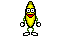Anyway, it generates a pseudo "landscape" using the wave spectrum analysis from fmod. You can drag your own song onto the exe and it will play it via command line, or just run the exe directly and it will play a song I wrote. I originally wrote this song on the guitar for a Tankwars game I made in QBasic, and "ported" it to Fruity Loops.I tried to add it as an attachment, but I guess it's too large. :p
Demo!

9
##### Challenges & Competitions / [PROCEDURAL] Landscape+Clouds
« on: April 18, 2008 »
Nicely done. Here's something I made, if you're interested. Mine is slow too. It has a little parallax scrolling thing going on that you can test with the left/right arrow keys.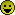10
##### Freebasic / Hi guys.
« on: February 05, 2008 »
A while back, some buds and I started working on this library called the FreeBASIC Extended Library. It has some pretty nice features, such as matrix/vector classes, a full sprite class(pretty much) and all kinds of string handling functions, sorting routines, etc... We're kind of shooting for FB's answer to boost. Anyway, here's a pretty nifty demo I put together that use FB's gfxlib, Newton physics lib and OpenGL. The full source code is in there, and fbext is open-source as well, so if you plan on making any games with FreeBASIC, don't forget about FBEXT!11
##### Freebasic / Here's a new glsl demo...
« on: May 31, 2007 »
It just runs a lens mapping shader on the current texture unit. I think it looks pretty cool.Here's the download and an extremely large screenshot.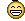http://file-pasta.com/d/1306.rar12
##### Freebasic / OpenGL Terrain generation/multi-texture demo.
« on: December 19, 2006 »
I made this so someone could learn how to do multi-texturing with terrain generation in OpenGL. It was actually part of another project of mine, but I just made a stand alone from different parts of it for this effort.By the way, sorry I've been posting so much the last few days. I've just been going through a lot of stuff I guess. hehehe

13
##### Freebasic / Fun with FB's gfxlib...
« on: December 19, 2006 »
I didn't post a screenshot because it requires the whole desktop.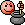Basically, it uses some of the new gfxlib functions to create a flag that scrolls around the screen. It kinda simulates a screensaver.

14
##### Freebasic / Here's a project from about a year ago...
« on: December 18, 2006 »
I don't plan on doing anything else to this because we have a better system now, but it's still useful code. I hope someone can get some use out of it!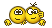Too big to attach to post...
http://qbnz.com/dr_davenstein/test.rar

15
##### Freebasic / Here's a .B3D(Blitz3D) loader...
« on: December 11, 2006 »
It was ported to FreeBASIC as an experiment. We're experimenting with different file formats for our Rogue3D game. When I say we, I mean rdc and I are working on a Rogue3D game. heheheÂI haven't fully tested any models with this yet, but it seems to be printing the correct vertices & stuff. I hope someone gets some use out of it.Code: [Select]
`Const False = 0, True = Not False#define Chunk_ID(a,b,c,d) ( ((a)) Or (b Shl(8)) Or (c Shl(16)) Or (d Shl(24)))#define Chunk_2String(_Chunk) Chr\$(_Chunk)+Chr\$(_Chunk Shr 8)+Chr\$(_Chunk Shr 16)+Chr\$(_Chunk Shr 24)#define ID_BB3DÂ  Chunk_ID( Asc("B"), Asc("B"), Asc("3"), Asc("D") )#define ID_TEXSÂ  Chunk_ID( Asc("T"), Asc("E"), Asc("X"), Asc("S") )#define ID_VRTSÂ  Chunk_ID( Asc("V"), Asc("R"), Asc("T"), Asc("S") )#define ID_ANIMÂ  Chunk_ID( Asc("A"), Asc("N"), Asc("I"), Asc("M") )#define ID_KEYSÂ  Chunk_ID( Asc("K"), Asc("E"), Asc("Y"), Asc("S") )#define ID_BRUSÂ  Chunk_ID( Asc("B"), Asc("R"), Asc("U"), Asc("S") )#define ID_TRISÂ  Chunk_ID( Asc("T"), Asc("R"), Asc("I"), Asc("S") )#define ID_MESHÂ  Chunk_ID( Asc("M"), Asc("E"), Asc("S"), Asc("H") )#define ID_BONEÂ  Chunk_ID( Asc("B"), Asc("O"), Asc("N"), Asc("E") )Declare Sub b3dExitChunk()Declare Sub Read_String( Byval File_Num As Uinteger, Byref tString As String )Declare Sub b3dSetFile( Byref file As Uinteger )Declare Function Dump_B3D() As IntegerDeclare Function b3dReadByte() As ByteDeclare Function b3dReadInt() As IntegerDeclare Function b3dReadFloat() As SingleDeclare Function b3dReadString() As StringDeclare Function b3dReadChunk() As StringDeclare Function b3dChunkSize() As IntegerDim Shared As Integer b3d_Stack(100), b3d_file, b3d_tosDim As Uinteger File_Num = Freefileb3dSetFile( File_Num )Open "Models/B3D/Test_Cylinder.b3d" For Binary As #File_NumÂ  Â  Dim As String ChunkÂ  Â  Dim As Integer VersionÂ  Â  If b3dReadChunk()<>"BB3D" Then Â  Â  Â  Â  Print "Invalid b3d file"Â  Â  End IfÂ  Â  Version = b3dReadInt()/100Â  Â  Print VersionÂ  Â  If Version>0 Then Â  Â  Â  Â  Print "Invalid b3d file version"Â  Â  End IfÂ  Â  Dump_B3D( )Close #File_NumSleepFunction Dump_B3D() As IntegerÂ  Â  Â  Â  Dim As String tname, ChunkÂ  Â  Dim As Integer flags, n_frames, n_keys, sz, frame, blend, n_texs, k, j, tex_idÂ  Â  Dim As Integer tc_sets, tc_size, n_verts, brush_idÂ  Â  Dim As Integer v0,v1,v2, n_tris, n_weights, vertex_idÂ  Â  Dim As Single fps = Any, fx = Any, weight = AnyÂ  Â  Dim As Single key_px = Any, key_py = Any, key_pz = AnyÂ  Â  Dim As Single key_sx = Any, key_sy = Any, key_sz = AnyÂ  Â  Dim As Single key_rw = Any, key_rx = Any, key_ry = Any, key_rz = AnyÂ  Â  Dim As Single x_pos = Any, y_pos = Any, z_pos = AnyÂ  Â  Dim As Single x_scl = Any, y_scl = Any, z_scl = AnyÂ  Â  Dim As Single w_rot = Any, x_rot = Any, y_rot = Any, z_rot = AnyÂ  Â  Dim As Single rot = Any, lfa = AnyÂ  Â  Dim As Single red = Any, grn = Any, blu = Any, alp = Any, shi = AnyÂ  Â  Dim As Single x = Any, y = Any, z = AnyÂ  Â  Dim As Single nx = Any, ny = Any, nz = AnyÂ  Â  Â  Â  Â  Â  Do While b3dChunkSize()Â  Â  Â  Â  Â  Â  Â  Â  chunk = b3dReadChunk()Â  Â  Â  Â  Print "Chunk: " & chunk & " size =" & b3dchunksize() Â  Â  Â  Â  Â  Â  Â  Â  Â  Â  Â  Â  Select Case chunkÂ  Â  Â  Â  Â  Â  Â  Â  Case "ANIM"Â  Â  Â  Â  Â  Â  Â  Â  Â  Â  Â  Â  flagsÂ  Â  = b3dReadInt()Â  Â  Â  Â  Â  Â  Â  Â  Â  Â  Â  Â  n_frames = b3dReadInt()Â  Â  Â  Â  Â  Â  Â  Â  Â  Â  Â  Â  fpsÂ  Â  Â  = b3dReadFloat()Â  Â  Â  Â  Â  Â  Print "Animation Flags/fps " & flags & "/" & fpsÂ  Â  Â  Â  Case "KEYS"Â  Â  Â  Â  Â  Â  Â  Â  Â  Â  Â  Â  flags=b3dReadInt()Â  Â  Â  Â  Â  Â  Â  Â  Â  Â  Â  Â  sz=4Â  Â  Â  Â  Â  Â  Â  Â  Â  Â  Â  Â  If flags And 1 Then sz=sz+12Â  Â  Â  Â  Â  Â  Â  Â  Â  Â  Â  Â  If flags And 2 Then sz=sz+12Â  Â  Â  Â  Â  Â  Â  Â  Â  Â  Â  Â  If flags And 4 Then sz=sz+16Â  Â  Â  Â  Â  Â  Â  Â  Â  Â  Â  Â  n_keys=b3dChunkSize()/szÂ  Â  Â  Â  Â  Â  Â  Â  Â  Â  Â  Â  If n_keys*sz=b3dChunkSize() ThenÂ  Â  Â  Â  Â  Â  Â  Â  Print "keys " & n_keysÂ  Â  Â  Â  Â  Â  Â  Â  Â  Â  Â  Â  Â  Â  Â  Â  'read all keys in chunkÂ  Â  Â  Â  Â  Â  Â  Â  Â  Â  Â  Â  Â  Â  Â  Â  While b3dChunkSize()Â  Â  Â  Â  Â  Â  Â  Â  Â  Â  Â  Â  Â  Â  Â  Â  Â  Â  Â  Â  frame = b3dReadInt()Â  Â  Â  Â  Â  Â  Â  Â  Â  Â  Â  Â  Â  Â  Â  Â  Â  Â  Â  Â  If flags And 1 ThenÂ  Â  Â  Â  Â  Â  Â  Â  Â  Â  Â  Â  Â  Â  Â  Â  Â  Â  Â  Â  Â  Â  Â  Â  key_px = b3dReadFloat()Â  Â  Â  Â  Â  Â  Â  Â  Â  Â  Â  Â  Â  Â  Â  Â  Â  Â  Â  Â  Â  Â  Â  Â  key_py = b3dReadFloat()Â  Â  Â  Â  Â  Â  Â  Â  Â  Â  Â  Â  Â  Â  Â  Â  Â  Â  Â  Â  Â  Â  Â  Â  key_pz = b3dReadFloat()Â  Â  Â  Â  Â  Â  Â  Â  Â  Â  End IfÂ  Â  Â  Â  Â  Â  Â  Â  Â  Â  If flags And 2 ThenÂ  Â  Â  Â  Â  Â  Â  Â  Â  Â  Â  Â  key_sx = b3dReadFloat()Â  Â  Â  Â  Â  Â  Â  Â  Â  Â  Â  Â  key_sy = b3dReadFloat()Â  Â  Â  Â  Â  Â  Â  Â  Â  Â  Â  Â  key_sz = b3dReadFloat()Â  Â  Â  Â  Â  Â  Â  Â  Â  Â  End IfÂ  Â  Â  Â  Â  Â  Â  Â  Â  Â  If flags And 4 ThenÂ  Â  Â  Â  Â  Â  Â  Â  Â  Â  Â  Â  key_rw = b3dReadFloat()Â  Â  Â  Â  Â  Â  Â  Â  Â  Â  Â  Â  key_rx = b3dReadFloat()Â  Â  Â  Â  Â  Â  Â  Â  Â  Â  Â  Â  key_ry = b3dReadFloat()Â  Â  Â  Â  Â  Â  Â  Â  Â  Â  Â  Â  key_rz = b3dReadFloat()Â  Â  Â  Â  Â  Â  Â  Â  Â  Â  End IfÂ  Â  Â  Â  Â  Â  Â  Â  WendÂ  Â  Â  Â  Â  Â  ElseÂ  Â  Â  Â  Â  Â  Â  Â  Print "Illegal number of keys!!!"Â  Â  Â  Â  Â  Â  End IfÂ  Â  Â  Â  Case "TEXS"Â  Â  Â  Â  Â  Â  While b3dChunkSize()Â  Â  Â  Â  Â  Â  Â  Â  tname = b3dReadString()Â  Â  Â  Â  Â  Â  Â  Â  flags = b3dReadInt()Â  Â  Â  Â  Â  Â  Â  Â  blend = b3dReadInt()Â  Â  Â  Â  Â  Â  Â  Â  x_pos = b3dReadFloat()Â  Â  Â  Â  Â  Â  Â  Â  y_pos = b3dReadFloat()Â  Â  Â  Â  Â  Â  Â  Â  x_scl = b3dReadFloat()Â  Â  Â  Â  Â  Â  Â  Â  y_scl = b3dReadFloat()Â  Â  Â  Â  Â  Â  Â  Â  rotÂ  Â = b3dReadFloat()Â  Â  Â  Â  Â  Â  Â  Â  Print tnameÂ  Â  Â  Â  Â  Â  WendÂ  Â  Â  Â  Case "BRUS"Â  Â  Â  Â  Â  Â  n_texs=b3dReadInt()Â  Â  Â  Â  Â  Â  'read all brushes in chunk...Â  Â  Â  Â  Â  Â  While b3dChunkSize()Â  Â  Â  Â  Â  Â  Â  Â  tname = b3dReadString\$()Â  Â  Â  Â  Â  Â  Â  Â  redÂ  Â = b3dReadFloat()Â  Â  Â  Â  Â  Â  Â  Â  grnÂ  Â = b3dReadFloat()Â  Â  Â  Â  Â  Â  Â  Â  bluÂ  Â = b3dReadFloat()Â  Â  Â  Â  Â  Â  Â  Â  alpÂ  Â = b3dReadFloat()Â  Â  Â  Â  Â  Â  Â  Â  shiÂ  Â = b3dReadFloat()Â  Â  Â  Â  Â  Â  Â  Â  blend = b3dReadInt()Â  Â  Â  Â  Â  Â  Â  Â  fxÂ  Â  = b3dReadInt()Â  Â  Â  Â  Â  Â  Â  Â  For k=0 To n_texs-1Â  Â  Â  Â  Â  Â  Â  Â  Â  Â  tex_id=b3dReadInt()Â  Â  Â  Â  Â  Â  Â  Â  NextÂ  Â  Â  Â  Â  Â  Â  Â  Print tnameÂ  Â  Â  Â  Â  Â  WendÂ  Â  Â  Â  Case "VRTS"Â  Â  Â  Â  Â  Â  flagsÂ  Â = b3dReadInt()Â  Â  Â  Â  Â  Â  tc_sets = b3dReadInt()Â  Â  Â  Â  Â  Â  tc_size = b3dReadInt()Â  Â  Â  Â  Â  Â  sz=12+tc_sets*tc_size*4Â  Â  Â  Â  Â  Â  If flags And 1 Then sz = sz + 12Â  Â  Â  Â  Â  Â  If flags And 2 Then sz = sz + 16Â  Â  Â  Â  Â  Â  n_verts=b3dChunkSize()/szÂ  Â  Â  Â  Â  Â  If n_verts*sz = b3dChunkSize() ThenÂ  Â  Â  Â  Â  Â  Â  Â  Print "vertex count: " & n_vertsÂ  Â  Â  Â  Â  Â  Â  Â  'read all verts in chunkÂ  Â  Â  Â  Â  Â  Â  Â  While b3dChunkSize()Â  Â  Â  Â  Â  Â  Â  Â  Â  Â  x = b3dReadFloat()Â  Â  Â  Â  Â  Â  Â  Â  Â  Â  y = b3dReadFloat()Â  Â  Â  Â  Â  Â  Â  Â  Â  Â  z = b3dReadFloat()Â  Â  Â  Â  Â  Â  Â  Â  Â  Â  Print x,y,zÂ  Â  Â  Â  Â  Â  Â  Â  Â  Â  If flags And 1 ThenÂ  Â  Â  Â  Â  Â  Â  Â  Â  Â  Â  Â  nx = b3dReadFloat()Â  Â  Â  Â  Â  Â  Â  Â  Â  Â  Â  Â  ny = b3dReadFloat()Â  Â  Â  Â  Â  Â  Â  Â  Â  Â  Â  Â  nz = b3dReadFloat()Â  Â  Â  Â  Â  Â  Â  Â  Â  Â  End IfÂ  Â  Â  Â  Â  Â  Â  Â  Â  Â  If flags And 2 ThenÂ  Â  Â  Â  Â  Â  Â  Â  Â  Â  Â  Â  red = b3dReadFloat()Â  Â  Â  Â  Â  Â  Â  Â  Â  Â  Â  Â  grn = b3dReadFloat()Â  Â  Â  Â  Â  Â  Â  Â  Â  Â  Â  Â  blu = b3dReadFloat()Â  Â  Â  Â  Â  Â  Â  Â  Â  Â  Â  Â  lfa = b3dReadFloat()Â  Â  Â  Â  Â  Â  Â  Â  Â  Â  End IfÂ  Â  Â  Â  Â  Â  Â  Â  Â  Â  'read tex coords...Â  Â  Â  Â  Â  Â  Â  Â  Â  Â  For j=1 To tc_sets*tc_sizeÂ  Â  Â  Â  Â  Â  Â  Â  Â  Â  Â  Â  Dim As Single tÂ  Â  Â  Â  Â  Â  Â  Â  Â  Â  Â  Â  t = b3dReadFloat()Â  Â  Â  Â  Â  Â  Â  Â  Â  Â  NextÂ  Â  Â  Â  Â  Â  Â  Â  WendÂ  Â  Â  Â  Â  Â  ElseÂ  Â  Â  Â  Â  Â  Â  Â  Print "Illegal number of vertices!!!"Â  Â  Â  Â  Â  Â  End IfÂ  Â  Â  Â  Case "TRIS"Â  Â  Â  Â  Â  Â  brush_id = b3dReadInt()Â  Â  Â  Â  Â  Â  sz=12Â  Â  Â  Â  Â  Â  n_tris = b3dChunkSize()/szÂ  Â  Â  Â  Â  Â  If n_tris * sz = b3dChunkSize() ThenÂ  Â  Â  Â  Â  Â  Â  Â  Print "Triangle count: " & n_trisÂ  Â  Â  Â  Â  Â  Â  Â  'read all tris in chunkÂ  Â  Â  Â  Â  Â  Â  Â  While b3dChunkSize()Â  Â  Â  Â  Â  Â  Â  Â  Â  Â  v0 = b3dReadInt()Â  Â  Â  Â  Â  Â  Â  Â  Â  Â  v1 = b3dReadInt()Â  Â  Â  Â  Â  Â  Â  Â  Â  Â  v2 = b3dReadInt()Â  Â  Â  Â  Â  Â  Â  Â  Â  Â  Â  Â  Â  Â  Â  Â  Â  Â  WendÂ  Â  Â  Â  Â  Â  ElseÂ  Â  Â  Â  Â  Â  Â  Â  Print "Illegal number of triangles!!!"Â  Â  Â  Â  Â  Â  End IfÂ  Â  Â  Â  Case "MESH"Â  Â  Â  Â  Â  Â  brush_id = b3dReadInt()Â  Â  Â  Â  Â  Â  Print "Brush ID " & brush_idÂ  Â  Â  Â  Case "BONE"Â  Â  Â  Â  Â  Â  sz=8Â  Â  Â  Â  Â  Â  n_weights = b3dChunkSize()/szÂ  Â  Â  Â  Â  Â  If n_weights * sz = b3dChunkSize() ThenÂ  Â  Â  Â  Â  Â  Â  Â  'read all weightsÂ  Â  Â  Â  Â  Â  Â  Â  Print "Weights " & n_weightsÂ  Â  Â  Â  Â  Â  Â  Â  While b3dChunkSize()Â  Â  Â  Â  Â  Â  Â  Â  Â  Â  vertex_id = b3dReadInt()Â  Â  Â  Â  Â  Â  Â  Â  Â  Â  weight = b3dReadFloat()Â  Â  Â  Â  Â  Â  Â  Â  WendÂ  Â  Â  Â  Â  Â  ElseÂ  Â  Â  Â  Â  Â  Â  Â  Print "Illegal number of bone weights!!!"Â  Â  Â  Â  Â  Â  End IfÂ  Â  Â  Â  Case "NODE"Â  Â  Â  Â  Â  Â  tname = b3dReadString\$()Â  Â  Â  Â  Â  Â  x_pos = b3dReadFloat()Â  Â  Â  Â  Â  Â  y_pos = b3dReadFloat()Â  Â  Â  Â  Â  Â  z_pos = b3dReadFloat()Â  Â  Â  Â  Â  Â  x_scl = b3dReadFloat()Â  Â  Â  Â  Â  Â  y_scl = b3dReadFloat()Â  Â  Â  Â  Â  Â  z_scl = b3dReadFloat()Â  Â  Â  Â  Â  Â  w_rot = b3dReadFloat()Â  Â  Â  Â  Â  Â  x_rot = b3dReadFloat()Â  Â  Â  Â  Â  Â  y_rot = b3dReadFloat()Â  Â  Â  Â  Â  Â  z_rot = b3dReadFloat()Â  Â  Â  Â  Â  Â  Print "name = " & tnameÂ  Â  Â  Â  End SelectÂ  Â  Â  Â  Â  Â  Â  Â  Dump_B3D()Â  Â  Â  Â  Â  'load the sub_chunksÂ  Â  Â  Â  b3dExitChunk()Â  Â  Â  Â  Â  Â  Â  Â  'exit this chunkÂ  Â  Â  Â  Â  Â  LoopÂ  Â  Return TrueEnd FunctionSub b3dSetFile( Byref file As Uinteger )Â  Â  Â  Â  b3d_tos=0Â  Â  Â  Â  b3d_file = fileEnd SubFunction b3dReadByte() As ByteÂ  Â  Dim As Byte rbyteÂ  Â  Get #b3d_file,, rbyteÂ  Â  Â  Â  Return rbyteEnd FunctionFunction b3dReadInt() As IntegerÂ  Â  Dim As Integer rIntÂ  Â  Get #b3d_file,, rIntÂ  Â  Return rIntEnd FunctionFunction b3dReadFloat() As SingleÂ  Â  Dim As Single rFloatÂ  Â  Get #b3d_file,, rFloatÂ  Â  Return rFloatEnd FunctionFunction b3dReadString() As StringÂ  Â  Dim As String TÂ  Â  Dim As Byte chÂ  Â  Â  Â  DoÂ  Â  Â  Â  Â  Â  Â  Â  ch=b3dReadByte()Â  Â  Â  Â  Â  Â  Â  Â  If ch=0 ThenÂ  Â  Â  Â  Â  Â  Return TÂ  Â  Â  Â  End IfÂ  Â  Â  Â  Â  Â  Â  Â  T+=Chr\$(ch)Â  Â  LoopEnd FunctionFunction b3dReadChunk() As StringÂ  Â  Dim As Integer k, szÂ  Â  Dim As String TagÂ  Â  Â  Â  For k=1 To 4Â  Â  Â  Â  Â  Â  Â  Â  tag+=Chr\$(b3dReadByte())Â  Â  NextÂ  Â  Â  Â  sz = b3dReadInt()Â  Â  Â  Â  b3d_tos+=1Â  Â  Â  Â  b3d_stack(b3d_tos) = Seek(b3d_file)+szÂ  Â  Â  Â  Return TagEnd FunctionSub b3dExitChunk()Â  Â  Seek b3d_file, b3d_stack(b3d_tos)Â  Â  Â  Â  b3d_tos-=1End SubFunction b3dChunkSize() As IntegerÂ  Â  Dim As Integer rintÂ  Â  Â  Â  rint = b3d_stack(b3d_tos)- Seek(b3d_file)Â  Â  Return rintEnd Function`

16
##### Freebasic / Here's a lens mapping thing...
« on: December 11, 2006 »
It's more of a tutorial than anything. It was a little less detailed before, but I decided to add a couple more effects to it. The code is pretty well commented, so if anyone wants easy lens mapping, here it is.17
##### Freebasic / Here's a simple glsl demo.
« on: July 15, 2006 »
It's a metablob demo using a glsl vertex shader. It wont run on old cards, so I wouldn't bother with it, if you do have an older card.
By the way, nice forum!ÂCode: [Select]
`Option Explicit Randomize Timer '\$Static '\$INCLUDE: "\gl\gl.bi" '\$INCLUDE: "\gl\glu.bi" '\$INCLUDE: "\gl\glext.bi" '\$INCLUDE: "\gl\glfw.bi" Const As Integer True = -1, False = Not True Type DisplayMode Â  Â  W As Uinteger Â  Â  H As Uinteger Â  Â  R_BITS As Uinteger Â  Â  G_BITS As Uinteger Â  Â  B_BITS As Uinteger Â  Â  A_BITS As Uinteger Â  Â  D_BITS As Uinteger Â  Â  S_BITS As Uinteger Â  Â  MODE As Uinteger Â  Â  GlVer As Zstring Ptr Â  Â  As Single FOVy, Aspect, zNear, zFar End Type Type Balls Â  Â  As Single R, G, B Â  Â  As Single x, Dx, y, Dy, Rad End Type Declare Sub Init_GL_Window( Display As DisplayMode ) Declare Sub Set_Ortho( Display As DisplayMode ) Declare Sub Drop_Ortho( Display As DisplayMode ) Declare Sub Init_Balls( Ball() As Balls, Display As DisplayMode) Const MAX_BALLS As Integer = 25 Dim As Integer Use_Shader = True Dim Ball(1 To Max_Balls) As Balls Dim Display As DisplayMode Init_GL_Window Display #Define Midx (Display.w \ 2) #Define Midy (Display.h \ 2) Dim glCreateShaderObjectARBÂ  Â As PFNglCreateShaderObjectARBPROCÂ  Â = Cptr(PFNGLCREATESHADEROBJECTARBPROC, glfwGetProcAddress( "glCreateShaderObjectARB" )) Dim glShaderSourceARBÂ  Â  Â  Â  Â As PFNglShaderSourceARBPROCÂ  Â  Â  Â  Â = Cptr(PFNGLSHADERSOURCEARBPROC, glfwGetProcAddress( "glShaderSourceARB" )) Dim glGetShaderSourceARBÂ  Â  Â  As PFNglGetShaderSourceARBPROCÂ  Â  Â  = Cptr(PFNGLGetSHADERSOURCEARBPROC, glfwGetProcAddress( "glGetShaderSourceARB" )) Dim glCompileShaderARBÂ  Â  Â  Â  As PFNGLCompileShaderARBPROCÂ  Â  Â  Â  = Cptr(PFNglCompileShaderARBPROC, glfwGetProcAddress( "glCompileShaderARB" )) Dim glDeleteObjectARBÂ  Â  Â  Â  Â As PFNGLDeleteObjectARBPROCÂ  Â  Â  Â  Â = Cptr(PFNglDeleteObjectARBPROC, glfwGetProcAddress( "glDeleteObjectARB" )) Dim glCreateProgramObjectARBÂ  As PFNglCreateProgramObjectARBPROCÂ  = Cptr(PFNglCreateProgramObjectARBPROC, glfwGetProcAddress( "glCreateProgramObjectARB" )) Dim glAttachObjectARBÂ  Â  Â  Â  Â As PFNglAttachObjectARBPROCÂ  Â  Â  Â  Â = Cptr(PFNglAttachObjectARBPROC, glfwGetProcAddress( "glAttachObjectARB" )) Dim glUseProgramObjectARBÂ  Â  Â As PFNglUseProgramObjectARBPROCÂ  Â  Â = Cptr(PFNglUseProgramObjectARBPROC, glfwGetProcAddress( "glUseProgramObjectARB" )) Dim glLinkProgramARBÂ  Â  Â  Â  Â  As PFNglLinkProgramARBPROCÂ  Â  Â  Â  Â  = Cptr(PFNglLinkProgramARBPROC, glfwGetProcAddress( "glLinkProgramARB" )) Dim glValidateProgramARBÂ  Â  Â  As PFNglValidateProgramARBPROCÂ  Â  Â  = Cptr(PFNglValidateProgramARBPROC, glfwGetProcAddress( "glValidateProgramARB" )) Dim glGetObjectParameterivARB As PFNglGetObjectParameterivARBPROC = Cptr(PFNglGetObjectParameterivARBPROC, glfwGetProcAddress( "glGetObjectParameterivARB" )) Dim glGetInfoLogARBÂ  Â  Â  Â  Â  Â As PFNglGetInfoLogARBPROCÂ  Â  Â  Â  Â  Â = Cptr(PFNglGetInfoLogARBPROC, glfwGetProcAddress( "glGetInfoLogARB" )) If glfwExtensionSupported( "GL_ARB_shader_objects" ) = 0 Then Â  Â  Print "Error: ARB shader objects extension not supported." Â  Â  Sleep 1000 Â  Â  End -1 End If If( glCreateShaderObjectARB = 0 ) Then Â  Â  Print "Error: glCreateShaderObjectARB not present." Â  Â  Sleep 1000 Â  Â  End -1 End If If( glCreateProgramObjectARB = 0 ) Then Â  Â  Print "Error: glCreateProgramObjectARB not present." Â  Â  Sleep 1000 Â  Â  End -1 End If If( glShaderSourceARB = 0 ) Then Â  Â  Print "Error: glShaderSourceARB not present." Â  Â  Sleep 1000 Â  Â  End -1 End If If( GL_ARB_shading_language_100 = 0 ) Then Â  Â  Print "Error: This program required GL_ARB_shading_language_100." Â  Â  Sleep 1000 Â  Â  End -1 End If Dim As Integer Line_Cnt Dim As String Shader_Text, tString Dim As Integer i, x, y, Scale = 8 Dim As IntegerÂ  Dist Dim As GlHandleARB Vertex_Shader, Shader_Program Dim As Gluint Shader_Compile_Success Read Line_Cnt For i = 1 To Line_Cnt Â  Â Read tString Â  Â Shader_Text + = tString + Chr( 13, 10 ) Next Dim As GLcharARB Ptr table(0) => { Strptr( Shader_Text ) } Vertex_Shader = glCreateShaderObjectARB( GL_VERTEX_SHADER ) glShaderSourceARB( Vertex_Shader, 1, @table(0), 0 ) glCompileShaderARB( Vertex_Shader ) glGetObjectParameterivARB(Vertex_Shader, GL_OBJECT_COMPILE_STATUS_ARB, @Shader_Compile_Success ) If Shader_Compile_Success = 0 Then Â  Â  Dim As Gluint infologsize Â  Â  glGetObjectParameterivARB( Vertex_Shader, GL_OBJECT_INFO_LOG_LENGTH_ARB, @infoLogSize) Â  Â  Dim As GlByte infolog(InfoLogSize) Â  Â  glGetInfoLogARB(Vertex_Shader, InfoLogSize, 0, @infoLog(0)) Â  Â  tString="" Â  Â  For i = 0 To InfoLogSize-1 Â  Â  Â  Â  tString+=Chr(InfoLog(i)) Â  Â  Next Â  Â  Â  Â  Print "Vertex Shader Infolog error message:" Â  Â  Print tString End If Shader_Program = GlCreateProgramObjectARB() glAttachObjectARB( Shader_Program, Vertex_Shader ) glLinkProgramARB( Shader_Program ) GlValidateProgramARB( Shader_Program ) glGetObjectParameterivARB( Shader_Program, GL_OBJECT_VALIDATE_STATUS_ARB, @Shader_Compile_Success ) If Shader_Compile_Success = 0 Then Â  Â  Beep Â  Â  Print "GLSL program failed to compile!" Â  Â  Sleep 1000 End If If GL_VERTEX_PROGRAM_POINT_SIZE_ARB = 0 Then Â  Â  Beep Â  Â  Print "GL_VERTEX_PROGRAM_POINT_SIZE_ARB required for program to run correctly!" Else Â  Â  GLEnable GL_VERTEX_PROGRAM_POINT_SIZE_ARB End If Set_Ortho( Display ) 'Drop_Ortho( Display ) Init_Balls( Ball(), Display ) Do Â  Â  Â  Â  If GlfwGetKey(GLFW_KEY_SPACE) Then Â  Â  Â  Â  Use_Shader Xor = True Â  Â  Â  Â  Do While GlfwGetKey(GLFW_KEY_SPACE) Â  Â  Â  Â  Â  Â  GlfwPollEvents() Â  Â  Â  Â  LoopÂ  Â  Â  Â  End If Â  Â  Â  Â  If Use_Shader Then Â  Â  Â  Â  glUseProgramObjectARB( Shader_Program ) Â  Â  Else Â  Â  Â  Â  glUseProgramObjectARB( False ) Â  Â  End If Â  Â  Â  Â  GlClear GL_COLOR_BUFFER_BIT Or GL_DEPTH_BUFFER_BIT Â  Â  Â  Â  For i = 1 To MAX_BALLS Â  Â  Â  Â  Ball(i).x += Ball(i).dx Â  Â  Â  Â  Ball(i).y += Ball(i).dy Â  Â  Â  Â  Â  Â  Â  Â  If Ball(i).x>360 Then Â  Â  Â  Â  Â  Â  Ball(i).dx -= (1+Rnd*.5) Â  Â  Â  Â  End If Â  Â  Â  Â  Â  Â  Â  Â  If Ball(i).x<280 Then Â  Â  Â  Â  Â  Â  Ball(i).dx += (1+Rnd*.5) Â  Â  Â  Â  End If Â  Â  Â  Â  Â  Â  Â  Â  If Ball(i).y>280 Then Â  Â  Â  Â  Â  Â  Ball(i).dy -= (1+Rnd*.5) Â  Â  Â  Â  End If Â  Â  Â  Â  Â  Â  Â  Â  If Ball(i).y<200 Then Â  Â  Â  Â  Â  Â  Ball(i).dy += (1+Rnd*.5) Â  Â  Â  Â  End If Â  Â  Â  Â  Â  Â  Next Â  Â  Â  Â  GlBegin GL_QUADS Â  Â  For y = Display.h To Scale Step -Scale Â  Â  Â  Â  For x = Scale To Display.w Step Scale Â  Â  Â  Â  Â  Â  Â  Â  Â  Â  Â  Â  Dim As Single R,G,B Â  Â  Â  Â  Â  Â  Dim As Integer Hits Â  Â  Â  Â  Â  Â  Â  Â  Â  Â  Â  Â  For i = 1 To MAX_BALLS Â  Â  Â  Â  Â  Â  Â  Â  Â  Â  Â  Â  Â  Â  Â  Â  Dist = ((y-Ball(i).y)^2 + (x-Ball(i).x)^2)/Ball(i).Rad Â  Â  Â  Â  Â  Â  Â  Â  Â  Â  Â  Â  Â  Â  Â  Â  If Dist<= Ball(i).Rad Then Â  Â  Â  Â  Â  Â  Â  Â  Â  Â  R += (Ball(i).Rad-Dist)/Ball(i).R Â  Â  Â  Â  Â  Â  Â  Â  Â  Â  G += (Ball(i).Rad-Dist)/Ball(i).G Â  Â  Â  Â  Â  Â  Â  Â  Â  Â  B += (Ball(i).Rad-Dist)/Ball(i).B Â  Â  Â  Â  Â  Â  Â  Â  Â  Â  Hits-=1 Â  Â  Â  Â  Â  Â  Â  Â  End If Â  Â  Â  Â  Â  Â  Â  Â  Â  Â  Â  Â  Â  Â  Next Â  Â  Â  Â  Â  Â  Â  Â  Â  Â  Â  Â  If Hits>0 Then Â  Â  Â  Â  Â  Â  Â  Â  R/=Hits Â  Â  Â  Â  Â  Â  Â  Â  G/=Hits Â  Â  Â  Â  Â  Â  Â  Â  B/=Hits Â  Â  Â  Â  Â  Â  End If Â  Â  Â  Â  Â  Â  GlEnable GL_BLEND Â  Â  Â  Â  Â  Â  GlBlendFunc GL_SRC_ALPHA, GL_ONE_MINUS_SRC_ALPHA Â  Â  Â  Â  Â  Â  GlColor4F R, G, B, 0 Â  Â  Â  Â  Â  Â  GlVertex3f X, Y, -Y / 500 Â  Â  Â  Â  Â  Â  GlColor4F R, G, B, 0 Â  Â  Â  Â  Â  Â  GlVertex3f X-Scale, Y, -Y / 500 Â  Â  Â  Â  Â  Â  GlColor4F R, G, B, 0 Â  Â  Â  Â  Â  Â  GlVertex3f X-Scale, Y-Scale, -( Y - Scale ) / 500 Â  Â  Â  Â  Â  Â  GlColor4F R, G, B, 0 Â  Â  Â  Â  Â  Â  GlVertex3f X, Y-Scale, -( Y - Scale ) / 500 Â  Â  Â  Â  Â  Â  Â  Â  Â  Â  Next Â  Â  Next Â  Â  GlEnd Â  Â  Â  Â  Â  Â  GlfwSwapBuffers Loop Until glfwGetKey( GLFW_KEY_ESC ) GlDeleteObjectArb( Vertex_Shader ) GlDeleteObjectArb( Shader_Program ) GlfwTerminate() End Sub Init_Balls( Ball() As Balls, Display As DisplayMode) Â  Â  Dim As Integer i Â  Â  Â  Â  For i = 1 To MAX_BALLS Â  Â  Â  Â  Ball(i).x = 320+Rnd*250 Â  Â  Â  Â  Ball(i).y = 240+Rnd*150 Â  Â  Â  Â  Ball(i).dx = 1+Rnd*10 Â  Â  Â  Â  Ball(i).dy = 1+Rnd*10 Â  Â  Â  Â  If Int(Rnd*10) = 5 Then Â  Â  Â  Â  Â  Â  Ball(i).dx = -Ball(i).dx Â  Â  Â  Â  Â  Â  If Int(Rnd*2) = 1 Then Ball(i).dy = -Ball(i).dy Â  Â  Â  Â  End If Â  Â  Â  Â  Â  Â  Â  Â  Ball(i).Rad = 64+Rnd*64 Â  Â  Â  Â  Â  Â  Â  Â  Ball(i).R = 64+Int(Rnd*255) Â  Â  Â  Â  Ball(i).G = 64+Int(Rnd*255) Â  Â  Â  Â  Ball(i).B = 64+Int(Rnd*255) Â  Â  Next Â  Â  End Sub Sub Set_Ortho( Display As DisplayMode ) Â  Â  GlMatrixMode( Gl_Projection ) Â  Â  GlPushMatrix Â  Â  GlLoadIdentity Â  Â  GlMatrixMode( Gl_Modelview ) Â  Â  GlPushMatrix Â  Â  GlLoadIdentity Â  Â  GlOrtho( 0, Display.W, 0, Display.H, -1, 1 ) End Sub Sub Drop_Ortho( Display As DisplayMode ) Â  Â  GlMatrixMode( Gl_Projection ) Â  Â  GlPopMatrix Â  Â  GlMatrixMode( Gl_Modelview ) Â  Â  GlPopMatrix End Sub Sub Init_GL_Window( Display As DisplayMode ) Â  Â  Display.WÂ  Â  Â = 640 Â  Â  Display.HÂ  Â  Â = 480 Â  Â  Display.R_BITS= 8 Â  Â  Display.G_BITS= 8 Â  Â  Display.B_BITS= 8 Â  Â  Display.A_BITS= 8 Â  Â  Display.D_BITS= 24 Â  Â  Display.S_BITS= 8 Â  Â  Display.MODEÂ  = GLFW_WINDOW Â  Â  Â  Â  If glfwInit() Then Â  Â  Â  Â  'Successful! Â  Â  ElseÂ  Â  Â  Â  Â  Â  Print "Failed to initialize GLFW!" Â  Â  Â  Â  Sleep 1000 Â  Â  Â  Â  End Â  Â  End If Â  Â  Â  Â  Â  Â  IfÂ  glfwOpenWindow( _ Â  Â  Display.WÂ  Â  Â , _ Â  Â  Display.HÂ  Â  Â , _ Â  Â  Display.R_BITS, _ Â  Â  Display.G_BITS, _ Â  Â  Display.B_BITS, _ Â  Â  Display.A_BITS, _ Â  Â  Display.D_BITS, _ Â  Â  Display.S_BITS, _ Â  Â  Display.MODEÂ  ) _ Â  Â  Then Â  Â  GlfwSwapInterval 1 Â  Â  Display.GlVer = glGetString(GL_VERSION) Else Â  Â  GlfwTerminate() Â  Â  End End If 'To check, use... glfwGetWindowParam() ' OpenGL specific stuff... glViewport 0, 0, Display.W, Display.H glMatrixMode GL_PROJECTIONÂ  Â  Â  Â  glLoadIdentityÂ  Â  Display.FOVy = 45.0 Display.Aspect = Display.W / Display.H Display.znear = 1 Display.zfar = 1000 gluPerspective Display.FOVy, Display.Aspect, Display.zNear, Display.zFar glShadeModel GL_SMOOTH glClearColor 0.0, 0.0, 0.0, 0.0 glClearDepth 1.0 glEnable GL_DEPTH_TEST glDepthFunc GL_LEQUALÂ  Â  glEnable GL_COLOR_MATERIAL glHint GL_PERSPECTIVE_CORRECTION_HINT, GL_NICEST glPolygonMode GL_FRONT_AND_BACK, GL_POINT glEnable GL_CULL_FACE End Sub Data 13 Data "void main(void)" Data "{" Data "gl_Position = gl_ModelViewProjectionMatrix * gl_Vertex;" Data "vec4 V = gl_ModelViewMatrix * gl_Vertex;" Data "gl_FrontColor = gl_Color;" Data "float ptSize = length(V);" Data "ptSize *= ptSize;" Data "gl_PointSize = (gl_Color * 10.0);" Data "if (gl_PointSize || 0 )" Data "{" Data "gl_FrontColor = gl_Color * (gl_PointSize/64);" Data "}" Data "}" `

Pages: